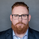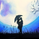Dilihat 16114
16114
This strategy is based off of Chris Moody's Vix Fix Indicator. I simply used his indicator and added some rules around it, specifically on entry and exits.

Rules:
• Enter upon a filtered or aggressive entry
• If there are multiple entry signals, allow pyramiding
• Exit when there is Stochastic RSI crossover above 80

This works great on a number of stocks. I am keeping a list of stocks with decent Profit Factors and clean equity curves here.

Possible ways to use this:
• Modify this script and setup alerts around the various entries
• Use as is with different stocks or currency pairs
• Modify entry / exit points to make it more profitable for even more symbols and currencies
```//@version=2
strategy("Vix FIX / StochRSI Strategy", pyramiding=9, initial_capital=10000, default_qty_type=strategy.percent_of_equity, default_qty_value=3, overlay=false)

///////////// Stochastic Slow
Stochlength = input(14, minval=1, title="lookback length of Stochastic")
StochOverBought = input(80, title="Stochastic overbought condition")
StochOverSold = input(20, title="Stochastic oversold condition")
smoothK = input(3, title="smoothing of Stochastic %K ")
smoothD = input(3, title="moving average of Stochastic %K")
k = sma(stoch(close, high, low, Stochlength), smoothK)
d = sma(k, smoothD)

///////////// RSI
RSIlength = input( 14, minval=1 , title="lookback length of RSI")
RSIOverBought = input( 70  , title="RSI overbought condition")
RSIOverSold = input( 30  , title="RSI oversold condition")
RSIprice = close
vrsi = rsi(RSIprice, RSIlength)

///////////// Double strategy: RSI strategy + Stochastic strategy

pd = input(22, title="LookBack Period Standard Deviation High")
bbl = input(20, title="Bolinger Band Length")
mult = input(2.0    , minval=1, maxval=5, title="Bollinger Band Standard Devaition Up")
lb = input(50  , title="Look Back Period Percentile High")
ph = input(.85, title="Highest Percentile - 0.90=90%, 0.95=95%, 0.99=99%")
new = input(false, title="-------Text Plots Below Use Original Criteria-------" )
sbc = input(false, title="Show Text Plot if WVF WAS True and IS Now False")
sbcc = input(false, title="Show Text Plot if WVF IS True")
new2 = input(false, title="-------Text Plots Below Use FILTERED Criteria-------" )
sbcFilt = input(true, title="Show Text Plot For Filtered Entry")
sbcAggr = input(true, title="Show Text Plot For AGGRESSIVE Filtered Entry")
ltLB = input(40, minval=25, maxval=99, title="Long-Term Look Back Current Bar Has To Close Below This Value OR Medium Term--Default=40")
mtLB = input(14, minval=10, maxval=20, title="Medium-Term Look Back Current Bar Has To Close Below This Value OR Long Term--Default=14")
str = input(3, minval=1, maxval=9, title="Entry Price Action Strength--Close > X Bars Back---Default=3")
//Alerts Instructions and Options Below...Inputs Tab
new4 = input(false, title="-------------------------Turn On/Off ALERTS Below---------------------" )
new5 = input(false, title="----To Activate Alerts You HAVE To Check The Boxes Below For Any Alert Criteria You Want----")
sa1 = input(false, title="Show Alert WVF = True?")
sa2 = input(false, title="Show Alert WVF Was True Now False?")
sa3 = input(false, title="Show Alert WVF Filtered?")
sa4 = input(false, title="Show Alert WVF AGGRESSIVE Filter?")

//Williams Vix Fix Formula
wvf = ((highest(close, pd)-low)/(highest(close, pd)))*100
sDev = mult * stdev(wvf, bbl)
midLine = sma(wvf, bbl)
lowerBand = midLine - sDev
upperBand = midLine + sDev
rangeHigh = (highest(wvf, lb)) * ph

//Filtered Bar Criteria
upRange = low > low and close > high
upRange_Aggr = close > close and close > open
//Filtered Criteria
filtered = ((wvf >= upperBand or wvf >= rangeHigh) and (wvf < upperBand and wvf < rangeHigh))
filtered_Aggr = (wvf >= upperBand or wvf >= rangeHigh) and not (wvf < upperBand and wvf < rangeHigh)

alert1 = wvf >= upperBand or wvf >= rangeHigh ? 1 : 0
alert2 = (wvf >= upperBand or wvf >= rangeHigh) and (wvf < upperBand and wvf < rangeHigh) ? 1 : 0
alert3 = upRange and close > close[str] and (close < close[ltLB] or close < close[mtLB]) and filtered ? 1 : 0
alert4 = upRange_Aggr and close > close[str] and (close < close[ltLB] or close < close[mtLB]) and filtered_Aggr ? 1 : 0

//Coloring Criteria of Williams Vix Fix
col = wvf >= upperBand or wvf >= rangeHigh ? lime : gray

isOverBought = (crossover(k,d) and k > StochOverBought) ? 1 : 0
isOverBoughtv2 = k > StochOverBought ? 1 : 0

plot(isOverBought, "Overbought / Crossover", style=line, color=red)

strategy.entry("Long", strategy.long)

if (isOverBought)
strategy.close("Long")

```brandonlongo0
@brandonlongo0, look at the code around aggressive and filtered long signals.
Jawabthanks for sharing such a great thing!
by the way, what period you use to trade with that strategy? did you try to mix VIX signals from different periods to make better Winning Percentage?
Jawab